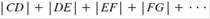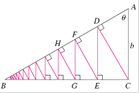### Create an Account

Home / Questions / A right triangle ABC is given with ∠A = θ and | AC | = b . CD is drawn perpendicular

# A right triangle ABC is given with ∠A = θ and | AC | = b . CD is drawn perpendicular

A right triangle ABC is given with ∠A = θ and |AC| = bCD is drawn perpendicular to ABDE is drawn perpendicular to BC, EF ⊥ AB, and this process is continued indefinitely as shown in the figure. Find the total length of all the perpendicularsin terms of b and θ.Apr 24 2020 View more View LessSubscribe To Get Solution